# Marston-Spangler Load Analysis Theory for Sewer Sanitary SystemReading time: 1 minute

Marston-Spangler theory for analysis of loads on sewer sanitary system pipe is established by Marston and it is applicable for most of the loading conditions that may encountered in site. Not only does this analysis approach based on the theory but also on experiments, and it is considered to be substantially reliable but conservative. Marston-Spangler theory for analysis of loads on sewer sanitary pipe is explored in this article.

## Marston-Spangler Load Analysis Theory for Sewer Sanitary System

Following topics regarding load analysis for sewer sanitary system is discussed:
• Marston-Spangler theory and assumptions

## Marston-Spangler Load Analysis Theory and Assumptions

The theory states that the load on the installed sewer sanitary pipe is equal to the weight of soil prism, which is termed as interior prism, on the pipe minus or plus the frictional shearing force transferred to the soil over the pipe by the trench wall side or exterior soil prisms on either side of interior prism. The direction and magnitude of the frictional force is dependent on the settlement of the prism over the pipe in relation to the neighboring soil prisms. Assumptions considered in this theory is that, the computed load equal to the load developed when maximum settlement is realized and Rankine theory is used to calculate lateral pressure which generates shearing force between the soil prism over the pipe and adjacent soil prisms. Finally, the general form of the equation of Marston-Spangler load analysis theory can be seen below:

W=CwB2 ->Equation-1

Where: W: vertical load per unit length acting on sewer pipe due to gravity soil loads C: dimensionless coefficient that combine the influence of the height of fill to trench width ratio, shearing force between interior prism and adjacent prisms and the direction and magnitude of interior prism settlement in relation to the adjacent prisms for embankment conditions. w: soil unit weight B: sanitary sewer trench breadth

Even though all factors required for the analysis of all types of sewer sanitary pipe placement conditions are included in the general form of the equation of Marston-Spangler theory, it is more suitable to categorize the placement conditions which may be encountered in the field, derive specific form of equations and generate graphs and tables for each type of installation condition. Trench, Negative-projecting embankment, Positive-projecting embankment and induced trench condition, which is a specific installation condition, are types of sewer sanitary pipe placement methods considered while Marston-Spangler loads analysis is conducted. These are explained in the following sections separately:

### Trench Installation Condition for Sewer Pipes

Trench condition is the case where the sanitary sewer pipe is placed in nearly narrow width trench excavated in undisturbed soil and covered with backfill materials to the original ground level as shown in Figure-1.Fig.1: Loads Imposed on Sewer Pipes in Trench Condition

In the trench installation method, the imposed load consists of two components which includes the load of the prism soil directly above the pipe and the shearing forces generated due to relative settlement of soil prism above the pipe and adjacent prisms. The direction of the shearing force created due to the relative settlement of interior prism in relation to the adjacent undisturbed soil is upward and consequently decrease the load of the prism to a certain degree. As a result, the force imposed on the pipe would be equal to the soil prism load minus shearing force. The weight of the prism is computed according to the following formula:

Wc=CdwBd2 -> Equation-2

Where: Wc: soil prism load w: backfill material density Bd: trench width at the top of the pipe Cd: is computed according to the following equation: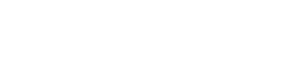Where: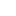: friction coefficient between interior prism and adjacent undisturbed soil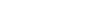H: height of backfill material above the pipe k: Rankine’s ratio of active lateral unit pressure to vertical unit pressure, and it can be computed using the following expression: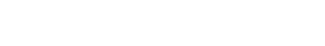Where: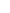: coefficient of internal friction of backfill material and it is always equal or greater than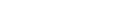. It should be known that equation-2 provides total load imposed on the sanitary sewer pipe. If the pipe is rigid, then it will support all the load calculate using equation-2. However, if the pipe is flexible and soil on both sides of the pipe is compacted to an extent that experience deformation under vertical load, that is smaller than the load under which the pipe itself is deformed, then backfill material on either side of the pipe are anticipated to support a portion of the vertical load. So, the equation used to estimate loads on the pipe would be as follow:

Wc=Cd wBc Bd -> Equation-5

Where the Bc is the outside width of the pipe. Finally, it is recommended to estimate the transition width of the trench because if the trench width is wider than the transition width for any reason, then it may be required to employ sanitary sewer pipe with greater strength. The transition width is the trench width at which the maximum vertical load is realized and the extra increase of trench width would not influence the load.

### Positive Projecting Embankment Condition for Sewer Pipes

In the case of positive projecting embankment condition, the pipe is covered above the original ground surface or the trench width is so large that the influence of trench wall friction does not exist and the top of the pipe is above the original ground surface. The load exerted on sanitary sewer pipe in positive projecting embankment condition is equal to the weight of soil prism above the pipe plus or minus the shearing force extended from the sewer pipe side upward into the embankment. When adequate embankment height is available, then it is likely that shearing force would not reach the top of the embankment but rather ends at some distance above the top of the pipe. The location at which shearing force ends is termed as plane of equal settlement as shown in Figure-2. Moreover, Figure-2 illustrates different types of settlements which involves settlement of natural ground adjacent to the pipe (sg), sewer pipe deflection (dc), settlement at the bottom of the pipe (sf), and compression of soil columns (sm) of height (pBc) that affect the load on the positive projecting sewer pipe. Furthermore, if the sanitary sewer pipe is pile supported in organic soil, it would be necessary to take down drag loads into consideration and the load imposed on the pipe is larger than that of interior prism over the pipe.Fig.2: Settlement which affects the Load Imposed on Positive Projecting Sanitary Sewer Pipe

Regarding fill loads which the pipe is subjected to in positive projecting embankment condition, it may be estimated using the following expression:

Wc=Cc wBc2 ->Equation 6

Where: Wc: load supported by sewer sanitary pipe w: backfill material density Bc: outside width of the pipe Cc: can be estimated using one of the following expressions: If plane of equal settlement ends at a distance above the pipe, the means H>He as can be observed in Figure-2, then the following formula is used to compute (Cc)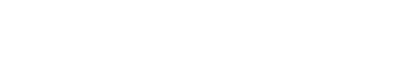Otherwise, the equation provided below should be applied to estimate (Cc):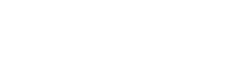To compute (He) in equation-7, the relationship between the deflection of the sewer sanitary pipe and relative settlement between interior soil prism and neighboring soil prisms should be considered. This relationship is called settlement ratio and may be evaluated as follow: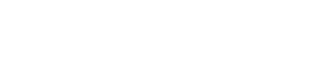Settlement ration design values have been established and advised to employ. These values can be found in Table-1.

### Table-1: Design Value for Settlement Ratio

 Sanitary sewer pipe type Soil condition Settlement ratio, rsd Rigid Rock or unyielding foundation +1 Rigid Ordinary foundation +0.5 to +0.8 Rigid Yielding foundation 0 to +0.5 Rigid Negative projecting installation -0.3 to +0.5 Flexible Poorly compacted fills on either side of pipe -0.4 to 0 Flexible Well compacted fill on either side of the pipe 0
The fill load exerted on the positive projecting pipe is affected by the multiplication of settlement ratio (rsd) times projection ratio (p). The projection ratio is equal to the vertical distance that the sanitary sewer pipe projects above the original ground surface divided by outside vertical height of the pipe (Bc'). The value of (Cc) can be estimated for different values [(H/Bc) rsd] and projection ratio (p) using Figure-3. It can be noticed that curved lines represent complete trench condition and projection condition whereas straight lines represent incomplete trench condition and projection condition. If the plane of equal settlement is located above the embankment, then the installation is termed as complete trench condition or complete projection condition according to the direction of the shearing force. However, If the plane of equal settlement is within embankment as shown in Figure-2, then the installation is incomplete trench condition or incomplete projection condition.Fig.3: Determination of Cc Coefficient for Positive Projecting Sewer Pipes

### Negative Projecting Embankment Condition for Sewer Pipes

In the case of negative embankment condition, the pipe is placed in a trench which is narrow compared with pipe size and trench depth. In this installation condition, the top of the pipe is below the original ground surface and the fill material over the pipe exceeds the original ground level surface as shown in Figure-4.Fig.4: Load on Negative Projection Sewer Pipes and Different Types of Settlement

When interior soil prism over the pipe experiences settlement, an upward shearing force is created which decrease the load imposed on the pipe. As the backfill material compressibility and negative projection ratio increases, the interior soil prism settlement increases. That is why materials such as sawdust and other material with similar property are frequently added to the soil directly above the sanitary sewer pipe to increase interior prism settlement. The load of fill material on the negative projecting sewer pipe is calculated using the following formula:

Wc=Cn wBd2 -> Equation-10

When the material inside sub-trench is compacted adequately, then equation-10 would be expressed as follow:

Wc=Cn wBd Bd' -> Equation-11

Where: Wc: load on the pipe w: backfill material density Bd: trench breadth Bd': average of trench width and outside diameter of the pipe Cn: can be estimated using one of the following expressions: If H>He as shown in Figure-4, then the following formula is used to compute (Cn)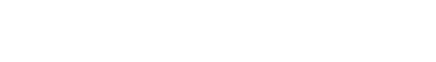Otherwise, the equation provided below should be applied to estimate (Cn):Similar to the positive projecting condition, it is required to specify the relationship between pipe deflection and relative settlement between interior prism and exterior prisms so as to evaluate (He). the relationship that is called settlement ratio is expressed as follow: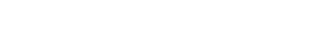Where: sd: the compression within the fill for the height of (p'Bd), where p' negative projection ratio which is equal to the vertical distance from the top of the pipe to the original ground surface at the time of installation divided by trench width. If the natural ground surface is not levelled, then it is required to consider average vertical distance from the top of sanitary sewer pipe to the both side of the trench Finally, table-1 provides recommended design values for settlement ratio for negative projecting condition. The value of (Cn) can be estimated for different values (rsd) and projection ratio (p) value of 0.5, 1. 1.5, and 2. Values falling between the provided projection ratio (p) should be found by interpolation.Fig.5: Cn values for Negative Projecting Condition Sewer Pipes

### Induced Trench Condition for Sewer Pipes

As can be noticed from the Figure-5, induced trench sewer pipe is initially placed as positive projecting sewer pipe. After that, an embankment, which is extended to a certain height above the ground surface is constructed over the pipe and properly compacted. Then, a trench is dug over the sewer pipe as shown in Figure-5, and filled with compressible material. Finally. The remaining part of the embankment is completed. The load on induced trench sewer pipe is estimated as follows:

Wc=Cn wBc2 -> Equation-15

Where: Bc: is the width of infill which is the same as the width of the pipe Cn: the same procedure used in the negative projecting condition is employed to estimate this coefficient.Fig.6: Induced Trench Sewer Pipe

Read More: Quality, Handling and Installation of Sewer Sanitary Pipes Design and Construction of Flexible Sewer Sanitary Pipes Special Construction of Sewer Sanitary Pipe System -Methods and Considerations How to Set Up Sewer Sanitary System Layout? Drains and Sewers Terms Definitions Direct Design of Concrete Pipes for Sewer Sanitary